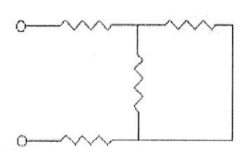# Problem: Each of the resistors in the circuit shown in the figure below has a resistance of 160.0 Ω. What is the equivalent resistance of the circuit? A) 160.0 Ω B) 400.0 Ω C) 480.0 Ω D) 640.0 Ω

###### FREE Expert Solution
81% (228 ratings)
###### Problem Details

Each of the resistors in the circuit shown in the figure below has a resistance of 160.0 Ω. What is the equivalent resistance of the circuit?

A) 160.0 Ω

B) 400.0 Ω

C) 480.0 Ω

D) 640.0 ΩFrequently Asked Questions

What scientific concept do you need to know in order to solve this problem?

Our tutors have indicated that to solve this problem you will need to apply the Combining Resistors in Series & Parallel concept. You can view video lessons to learn Combining Resistors in Series & Parallel. Or if you need more Combining Resistors in Series & Parallel practice, you can also practice Combining Resistors in Series & Parallel practice problems.

How long does this problem take to solve?

Our expert Physics tutor, Douglas took 5 minutes and 2 seconds to solve this problem. You can follow their steps in the video explanation above.

What professor is this problem relevant for?

Based on our data, we think this problem is relevant for Professor Freedman's class at UCSB.